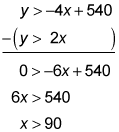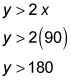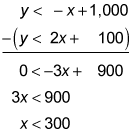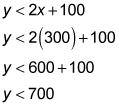##### SAT: 1,001 Practice Questions For DummiesIf you encounter a question on the SAT Math exam that deals with systems of inequalities, you can solve it using the same approach as for a system of equations.

The following practice questions ask you to find the minimum and maximum possible values of a y-coordinate in a given solution set.

## Practice questions

1. In the xy-plane, if a point with the coordinates (c, d) lies in the solution set of this system of inequalities, what is the minimum possible value of d? y > –4x + 540 y > 2x A. Slightly below 90 B. Slightly above 90 C. Slightly below 180 D. Slightly above 180
2. In the xy-plane, if a point with the coordinates (e, f) lies in the solution set of this system of inequalities, what is the maximum possible value of f? y < –x + 1,000 y < 2x + 100 A. Slightly below 300 B. Slightly above 300 C. Slightly below 700 D. Slightly above 700

1. The correct answer is Choice (D). Combine the inequalities to find the (x, y) values of where the lines cross. First subtract the second inequality from the first inequality; then solve for x:Now plug in 90 for x in the second inequality to solve for y:The (x, y) values of where the lines cross are (90, 180). Because each inequality has a y that's greater than the expression with the x, anything above the lines is within the solution set; d represents the y-value of where the lines cross, so the answer is slightly above 180.
2. The correct answer is Choice (C). Combine the inequalities to find the (x, y) values of where the lines cross. First subtract the second inequality from the first inequality; then solve for x:Now plug in 300 for x in the second inequality to solve for y:The (x, y) values of where the lines cross are (300, 700). Because each inequality has a y that's less than the expression with the x, anything below the lines is within the solution set; f represents the y-value of where the lines cross, so the answer is slightly below 700.